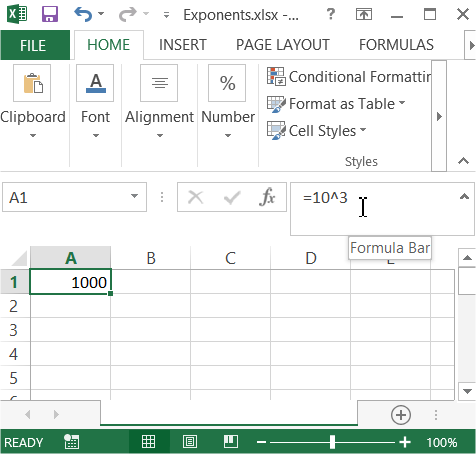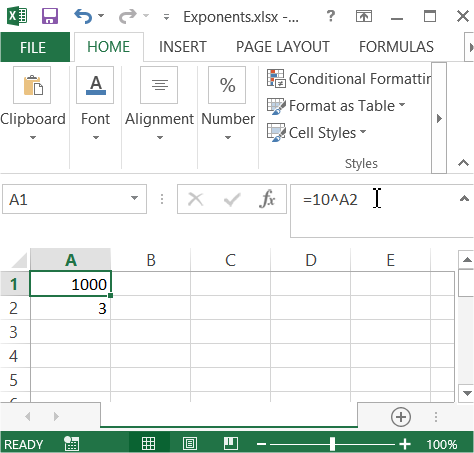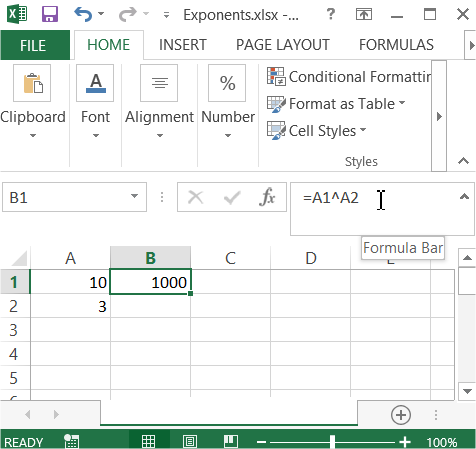### Exponents in Excel

In this Excel lesson you will teach yourself how to use exponents.

Exponent means how many times the number will be multiplied by itself. For example 103 (10 to the 3rd power) equals 10 * 10 * 10 which is 1 000.

The power of exponent in Excel is a carot symbol (SHIFT + 6 keyboard shortcut) which is ^. So you will write 10 to the 3rd power in Excel by 10^3.

To type exponents in Excel just use carot.

In cell you can just write =10^3.Of course there is a possibility to use cell’s address like =10^A2Also =A1^3 is possible.And =A1^A2 as well.Excel offers POWER function for exponents calculations. Write =POWER(10,3) to calculate our example.You can use carot or POWER function. Choose one which you prefer.

Math’s is much easier with Excel.

## Template

```Further reading: